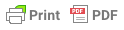# Formatting mathematical equations and statisticsIt is the author’s responsibility to ensure the correctness of mathematical equations; this will not be checked during the editorial process. It is therefore important to review the typeset proofs carefully to ensure that errors were not introduced during the editorial process and typesetting of the equations.

Below are some examples of properly formatted equations and statistical values:

t(75) = 2.14

R2 = .269

#p < .10, *p < .05, **p < .01, ***p < .001

a = -133.8 + 1.14 × b

## Equations and statistics requirements for final manuscript delivery

The following requirements must be met before the final delivery of your manuscript.

1. Place a space on either side of mathematical symbols (= , + , – , < , > , × , / , etc.).

2. Italicise variables (e.g., R2, Pearson’s r, and x = y + 2). (If variables are in an italicised heading, do not use reverse italics; e.g., do not write R2 like this.)

3. N = full sample; n = subsample

4. Use a hyphen with no space to indicate a negative numeral (e.g., -43).

5. Use an en dash with a space on either side for a subtraction sign (e.g., 5 – 3 = 2).

6. The multiplication sign can be found with the “insert symbol” function in Word – it looks like this: × (do not use an asterisk [*] or a lower- or uppercase “x” for a multiplication sign).

7. Use decimal points (periods), not commas, to express decimals (e.g., 1.05).

8. Use commas, not periods or spaces, to separate thousands (e.g., 1,000 and 2,000,000).

9. Use the following abbreviations without periods: minimum = min; maximum = max; standard deviation = std dev; standard errors = std errors